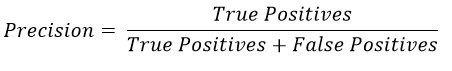Classification

Logistic Regression

Machine Learning

December 31, 2020

# What is Precision?

Precision measures the number of True Positives out of the Total number of positive cases predicted by the model.Precision is used to check the reliability of the model. If the precision of our model were low, we wouldn’t rely too much on the results on the model and would:

• Build a new model with higher precision. This step would be used if the recall of the model is also low
• Include other checks in the screening process that further filter out the model results. This step would be taken if the model has high Recall.

by : Monis Khan

Quick Summary:

Precision measures the number of True Positives out of the Total number of positive cases predicted by the model. Precision is used to check the reliability of the model. If the precision of our model were low, we wouldn’t rely too much on the results on the model and would: Build a new model with […]